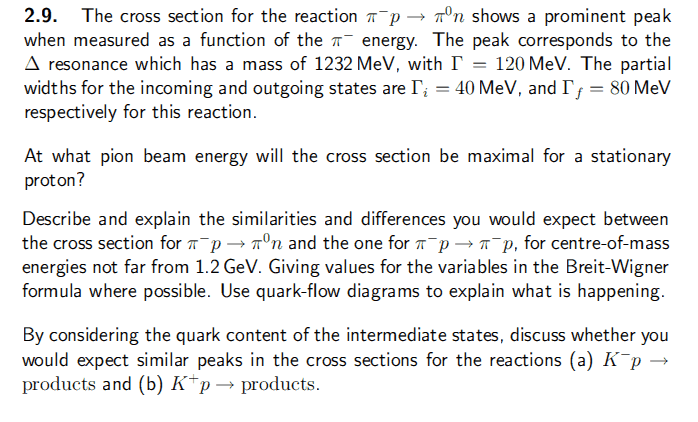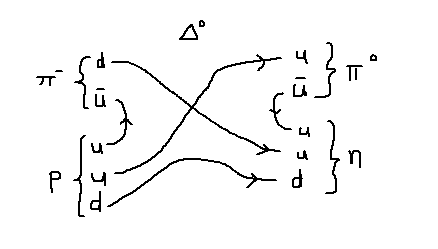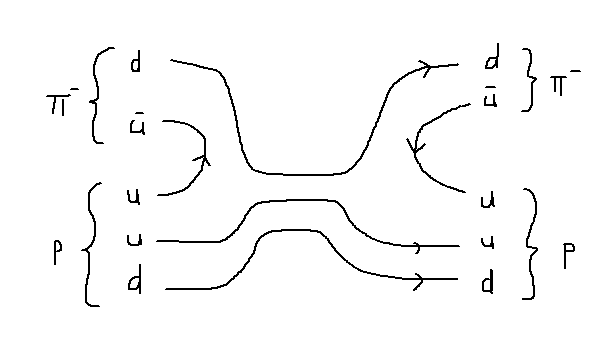# Quark Scattering and Quark Flow Diagrams

## Homework Statement

(a) Find energy of incoming beam that creates highest cross section
(b) What are the differences in the two reactions, using quark diagrams?
(c) What would the peaks of the two reactions be like?## The Attempt at a Solution

Part(a)
[/B]
Cross section is given by Breit-Wigner formula:

$$\sigma = \frac{\pi}{k^2} \frac{\Gamma_i \Gamma_f}{ (E-E_0)^2 + \frac{\Gamma^2}{4}}$$

Using centre of mass frames and 4-momentum:

I find ##E_{\pi} = \frac{E_0^2 - m_{\pi}^2 - m_p^2}{2m_p} = 329.3~MeV##.

Part(b)

First reaction is given by: ## \pi^{-} + p \rightarrow \pi^0 + n ## and quark flow diagram is shown:The second reaction is given by: ##\pi^{-} + p \rightarrow \pi^{-} + p## and quark flow diagram is shown:I'm not sure how the reaction would be different. I'm guessing reaction 1 would be more favourable, since the products are lighter?